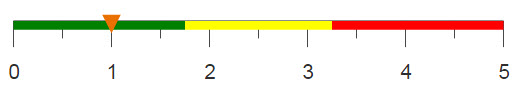# Converting names to initials

Using string manipulations, you can convert a column containing names to a column containing initials.

## Difficulty## Objective

You have a column containing the first and last names of all the people in a particular group. You would like to create a column containing just the initials of each person.

## Solution

```<base table="pub.doc.retail.altseg.stores"/>
<willbe name="first" value="taketo(manager;' ')"/>
<willbe name="second" value="dropto(manager;' ')"/>
<willbe name="first_initial" value="first(first;1)"/>
<willbe name="second_initial" value="first(second;1)"/>
<willbe name="initials" label="Initials"
value="splice(first_initial second_initial;'')"/>
<colord hide="first,second,first_initial,second_initial"/>```

## Alternate solution

```<base table="pub.doc.retail.altseg.stores"/>
<willbe name="initials" label="Initials"
value="splice(first(manager;1) first(dropto(manager;' ');1);'')"/>```

## Discussion

When it is not necessary to retain a complete string within a column, converting to initials can be useful and simplify your data table. In this solution, string functions are used to extract the first letter from the first and last name of a person. These letters are then combined to create a column containing just the person's initials.

In the main solution, the first and last names are initially split into two separate columns, and then two additional columns are created to hold the first letter from those columns. The initials columns is then created using the splice(X;Y) function, which combines the columns containing the first and second initials. Finally, the <colord> operation hides the intermediate columns that were used in the transformation.

The alternate solution uses the same functions but combines them into one line to simplify the code and avoid the need to create unnecessary columns. This solution also avoids using taketo(X;Y) by simply using the first letter in the original column that contained the name.

## Further reading

If you would like to learn more about the functions and operations discussed in this recipe, click on the links below:

taketo(X;Y)

dropto(X;Y)

splice(X;Y)